# Theremino

A theremin made out of two ultrasonic sensors.

BeginnerShowcase (no instructions)3,239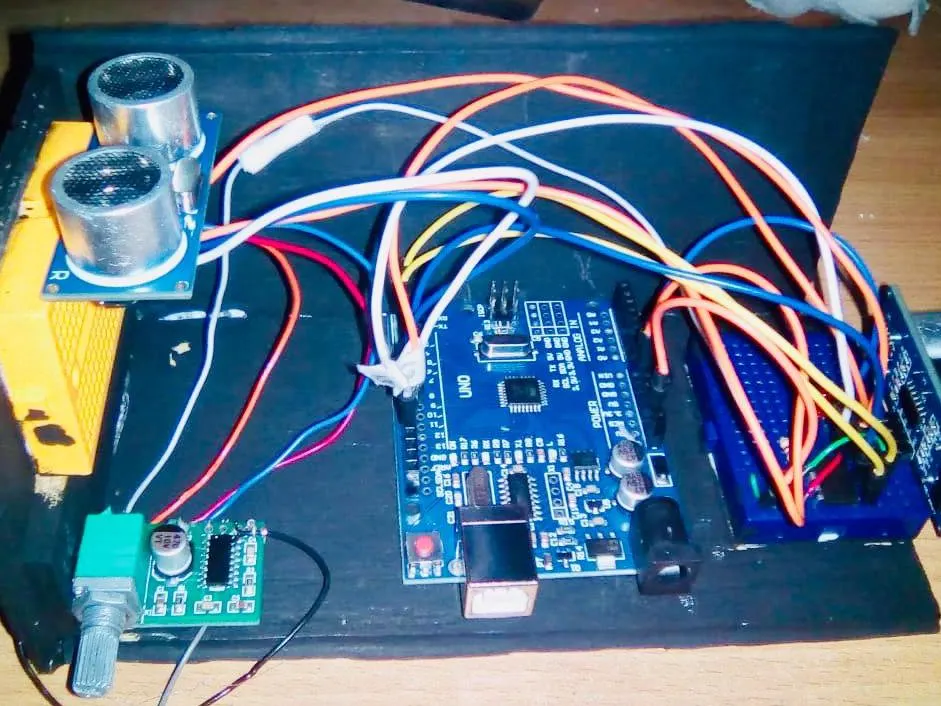## Things used in this project

### Hardware componentsArduino UNO & Genuino UNO
×1Ultrasonic Sensor - HC-SR04 (Generic)
×2×3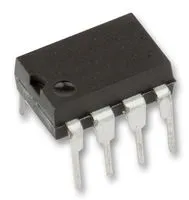Non Volatile Digital Potentiometer, 10 kohm we use a x9c103 model
×1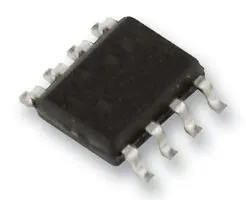Operational Amplifier, Op Amp + Comparator + Reference optional
×1Jumper wires (generic)
×1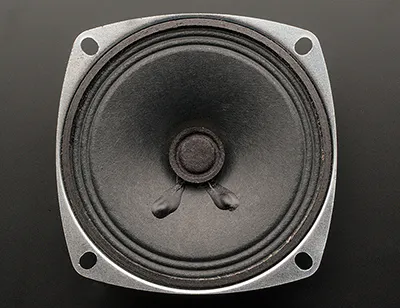Speaker: 3W, 4 ohms
×1LED (generic)
×1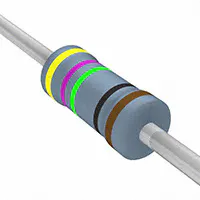Resistor 475 ohm
×1

### Hand tools and fabrication machinesHot glue gun (generic)

## Schematics

### Theremin circuit

Is an schematic for the Arduino theremin. Just copy the set up.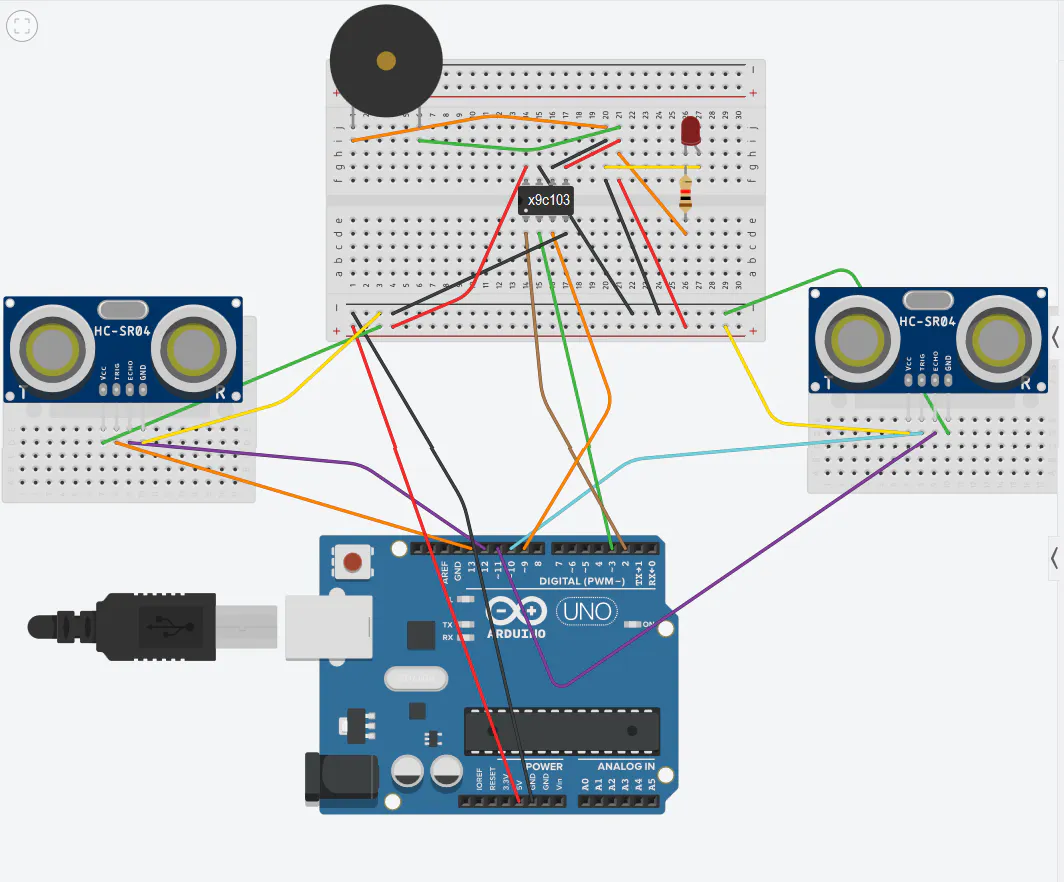## Code

### Arduino Theremin

Arduino
Is a code for the arduino theremin
```//In this project we create a theremin with two ultrasonic sensors, one for volume and another for tone. We use the digital potentiometer X9C103P.
/*
* Potentiometer conecctions:
* 1 - INC - Arduino pin 2
* 2 - U/D - Arduino pin 3
* 3 - VH  - 5V
* 4 - VSS - GND
* 5 - VW  - Output
* 6 - VL  - GND
* 7 - CS  - Arduino pin 4
* 8 - VCC - 5V
*
*/
//Note: we include two libraries: NewPing and TonePlayer because if we use the library NewPing with a tone function, the sketch doesnot compile.
#include <NewPing.h>
#include <TonePlayer.h>
#define MIN_DISTANCIA 35
TonePlayer tone1(TCCR1A, TCCR1B, OCR1AH, OCR1AL, TCNT1H, TCNT1L);

NewPing sonar(10, 11, 35); //The first and the second number are the pins of the sensor of volume, the third is the maximum distance

// name pins for sensors and the potentiometer
int echo = 12;
int trigger = 13;
int INC = 2;
int UD = 3;
int distancia;
int actual, anterior;
// necessary variables
unsigned long tiempoRespuesta;
float distancia2;
float tono1;

void setup() {
// set all the pins
Serial.begin(9600); //use of monitor serie for check the distance
pinMode(trigger, OUTPUT); //Tone sensor
pinMode(echo, INPUT); //Tone sensor
pinMode(2, OUTPUT); //Potentiometer
pinMode(3, OUTPUT); //Potentiometer
//pinMode (speaker, OUTPUT);

for(int j=0; j<90; j++){ //As we do not know in what value the potentiometer is, we place the value at the minimum
decremento();
}

}

void loop() {

digitalWrite(trigger, HIGH);
delayMicroseconds(10);   // Pulse of ten microseconds
digitalWrite(trigger, LOW);
tiempoRespuesta = pulseIn(echo, HIGH);  // Echo of the pulse
distancia2 = tiempoRespuesta/58;  //Calculation of the distance in centimeters

if (distancia2 < MIN_DISTANCIA) {
//Conversion of distance into a value for a sound
tono1 = 50.0*pow(2,(distancia2/12.0));  //pow alculates the value of a number raised to a power
pinMode(9, OUTPUT);
tone1.tone(tono1);  //Generates the tone with the previous value (220 Hz)
delay(10);
}

distancia = sonar.ping_cm(); //Calculation of the distance in centimeters (with the library NewPing)
Serial.print(distancia); //Check the distance
//Change the value "distancia", to values that are useful for the rise and fall of the potentiometer
actual = map(distancia, 0, 35, 0, 200);

//Compares the mapped values to raise or lower the potentiometer
if(actual > anterior){
for(int i = 0; i< (actual - anterior); i++){
decremento();
}
}

else{
for(int i = 0; i <= (anterior - actual); i++)
{
aumento();
}
}

anterior = actual; //The value is updated with each loop
delay(1);

}

//The U/D input controls the direction of the wiper movement and whether the counter is incremented or decremented
//The INC input is negative-edge triggered. Toggling INC will move the wiper and either increment or decrement the counter in the direction indicated by the logic level on the U/D input.
//The next two functions produce these toggling in the two directions (increment, decrement)

void aumento(){ //aumento == increment
digitalWrite(UD, HIGH);
digitalWrite(INC, LOW);
digitalWrite(INC, HIGH);
delay(10);
digitalWrite(INC, LOW);
}

void decremento(){ //decremento == decrement
digitalWrite(UD, LOW);
digitalWrite(INC, LOW);
digitalWrite(INC, HIGH);
delay(10);
digitalWrite(INC, LOW);
}
```

## Credits

### opus9

0 projects • 5 followers